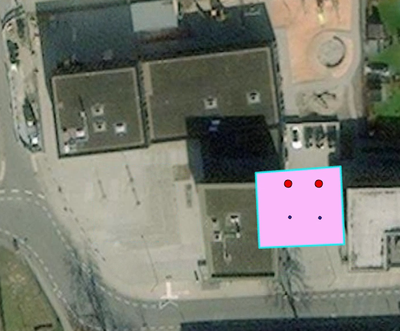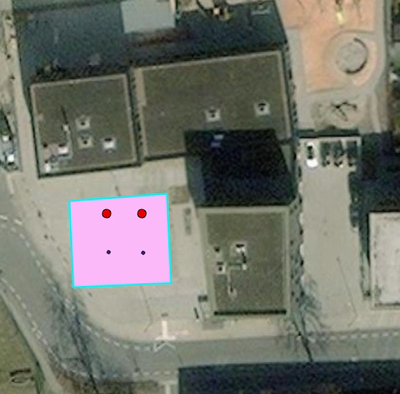# Attribute Rule for Polygon Movement with Intersecting Feature Constraints

508
3
01-25-2023 12:39 AMOccasional Contributor

Hey fellow-esris,

I hope all is well with you. I've been working on a project and I'm trying to set up an attribute rule (constraint) for a polygon feature class that would only allow the polygon to be moved if all intersecting features are moved with it. But I'm a bit stuck and could use some help. This is the code I got so far:

var targetAssemblyNumbers = '(2, 3, 900)'; // Assemblies which must be within stationboundaries
var targetDeviceNumbers = '(1, 2, 4, 6, 19)'; // Devices which must be within stationboundaries
var targetJunctionNumbers = '(5,50,950)'; // Junctions which must be within stationboundaries

var assemblies = FeatureSetByName(\$datastore, 'PipelineAssembly', ['globalid'], true)
var devices = FeatureSetByName(\$datastore, 'PipelineDevice', ['globalid'], true)
var junctions = FeatureSetByName(\$datastore, 'PipelineJunction', ['globalid'], true)

var targetAssemblies = Filter(assemblies, Concatenate(['ASSETGROUP', 'IN', targetAssemblyNumbers], ' '))
var targetDevices = Filter(devices, Concatenate(['ASSETGROUP', 'in', targetDeviceNumbers], ' '))
var targetJunctions = Filter(junctions, Concatenate(['ASSETGROUP', 'in', targetJunctionNumbers], ' '))

var preCounter = 0

preCounter += count(Intersects(targetAssemblies, Geometry(\$originalFeature)))
preCounter += count(Intersects(targetDevices, Geometry(\$originalFeature)))
preCounter += count(Intersects(targetJunctions, Geometry(\$originalFeature)))

var postCounter = 0

postCounter += count(Intersects(targetAssemblies, Geometry(\$feature)))
postCounter += count(Intersects(targetDevices, Geometry(\$feature)))
postCounter += count(Intersects(targetJunctions, Geometry(\$feature)))

if(preCounter != postCounter){
return false;
} else return true;

Any ideas or tips would be greatly appreciated.

1 Solution

Accepted SolutionsbyMVP Frequent Contributor

I believe that you can't accomplish this with a Constraint rule. The problem is that you have to know the old and new positions not only of the \$feature, but of the intersecting features as well. AFAIK, there's no way to get the old geomtries of the intersecting features.

I would do this with a Calculation Rule instead. In Calculation rules, you can leave the field empty and return a dictionary. With this dictionary, you can declare edits to be made to other tables. For a documentation of this dictionary, see here: Attribute rule dictionary keywords—ArcGIS Pro | Documentation

Other things:

• If the geometry isn't changed, there is no need to load all those featuresets. Abort early.
• Instead of writing the same code for multiple featuresets, use a for loop.
``````// Calculation Attribute Rule on the poylgon FC
// field: empty!
// triggers: Update
// Exclude from Application Evaluation

var old_geo = Geometry(\$originalfeature)
var new_geo = Geometry(\$feature)
// if the geometry didn't change, we don't need to update the points
if(Equals(old_geo, new_geo)) { return }
// if the geometry changed, but it wasn't a simple move (eg you edited some vertices), we can't update the points
if(Area(old_geo) - Area(new_geo) > 0.0001) { return }

// calculate the coordinate change
var old_centroid = Centroid(old_geo)
var new_centroid = Centroid(new_geo)
var dx = new_centroid.x - old_centroid.x
var dy = new_centroid.y - old_centroid.y

// load and filter the associated point fcs
// the dictionary keys have to be the classnames!
var fcs = {
"TestPoints": Filter(FeaturesetByName(\$datastore, "TestPoints", ["ObjectID"], true), "IntegerField IN (1, 2, 3)"),
"Stations": Filter(FeaturesetByName(\$datastore, "Stations", ["ObjectID"], true), "TextField IN ('a', 'b', 'c')"),
}

// loop over the associated fcs
var edits = []
for(var fc_name in fcs) {
var fc = fcs[fc_name]
// create the update array
// get the points that intersected the \$originalfeature
var i_fc = Intersects(fc, old_geo)
// loop over those points
for(var f in i_fc) {
// calculate the new point geometry
var f_geo = Geometry(f)
var new_x = f_geo.x + dx
var new_y = f_geo.y + dy
var new_f_geo = Point({x: new_x, y: new_y, spatialReference: f_geo.spatialReference})
// append that new geometry to the update array
}
}
// return the edits
return {edit: edits}``````

All you have to do is move the polygon, the rule will move the associated points for you.Have a great day!
Johannes
3 RepliesbyEsri Frequent Contributor

What is not working?  Why are you checking \$feature, if you only care if the original geometry did not intersect a feature?

I would suggest adding an exit early code if the geometry did not move.  This rule fires for every edit

You also can exit early once you find the first intersecting feature, since your rule is all features need to be moved.  Once you find one, who cares if you find another

You use a loop to clean the code up some.

``````if (Equals(\$feature,\$OriginalFeature)){
return true;
}

var subs_fs = [
['(2, 3, 900)',FeatureSetByName(\$datastore, 'PipelineAssembly', ['globalid'], true)],
['(1, 2, 4, 6, 19)',FeatureSetByName(\$datastore, 'PipelineDevice', ['globalid'], true)],
['(5,50,950)',FeatureSetByName(\$datastore, 'PipelineJunction', ['globalid'], true)]
];
for (var i in subs_fs){
var filt_fs = Filter(subs_fs[i], Concatenate(['ASSETGROUP', 'IN', subs_fs[i]], ' '));
var cnt = Count(Intersects(filt_fs, Geometry(\$originalFeature)));
if (cnt > 0){
return false;
}
}

return true;``````byMVP Frequent Contributor

I believe that you can't accomplish this with a Constraint rule. The problem is that you have to know the old and new positions not only of the \$feature, but of the intersecting features as well. AFAIK, there's no way to get the old geomtries of the intersecting features.

I would do this with a Calculation Rule instead. In Calculation rules, you can leave the field empty and return a dictionary. With this dictionary, you can declare edits to be made to other tables. For a documentation of this dictionary, see here: Attribute rule dictionary keywords—ArcGIS Pro | Documentation

Other things:

• If the geometry isn't changed, there is no need to load all those featuresets. Abort early.
• Instead of writing the same code for multiple featuresets, use a for loop.
``````// Calculation Attribute Rule on the poylgon FC
// field: empty!
// triggers: Update
// Exclude from Application Evaluation

var old_geo = Geometry(\$originalfeature)
var new_geo = Geometry(\$feature)
// if the geometry didn't change, we don't need to update the points
if(Equals(old_geo, new_geo)) { return }
// if the geometry changed, but it wasn't a simple move (eg you edited some vertices), we can't update the points
if(Area(old_geo) - Area(new_geo) > 0.0001) { return }

// calculate the coordinate change
var old_centroid = Centroid(old_geo)
var new_centroid = Centroid(new_geo)
var dx = new_centroid.x - old_centroid.x
var dy = new_centroid.y - old_centroid.y

// load and filter the associated point fcs
// the dictionary keys have to be the classnames!
var fcs = {
"TestPoints": Filter(FeaturesetByName(\$datastore, "TestPoints", ["ObjectID"], true), "IntegerField IN (1, 2, 3)"),
"Stations": Filter(FeaturesetByName(\$datastore, "Stations", ["ObjectID"], true), "TextField IN ('a', 'b', 'c')"),
}

// loop over the associated fcs
var edits = []
for(var fc_name in fcs) {
var fc = fcs[fc_name]
// create the update array
// get the points that intersected the \$originalfeature
var i_fc = Intersects(fc, old_geo)
// loop over those points
for(var f in i_fc) {
// calculate the new point geometry
var f_geo = Geometry(f)
var new_x = f_geo.x + dx
var new_y = f_geo.y + dy
var new_f_geo = Point({x: new_x, y: new_y, spatialReference: f_geo.spatialReference})
// append that new geometry to the update array
}
}
// return the edits
return {edit: edits}``````

All you have to do is move the polygon, the rule will move the associated points for you.Have a great day!
JohannesOccasional Contributor

Thanks for the input. In the end i used both your script-ideas. The first one to not allow the polygon be edited so that intersecting features would be out of the polygon boundaries. and the second one to still be able to move the features with the whole polygon structure. Thanks four your help!